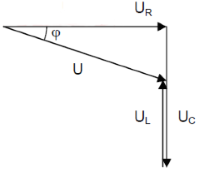# RLC series circuit calculation

Calculator and formulas for calculating the voltage and power of an RLC series circuit

## Calculate series circuit of the inductor, capacitor and resistor

The calculator calculates the voltages, powers, currents, impedance and reactance in the series circuit of a resistor of a inductor and a capacitor.

Calculate RLC series circuit

 Input Inductance L H mH µH nH Capacitor C F mF µF nF pF Frequency f Hz kHz MHz GHz Resistance R mΩ Ω kΩ MΩ Voltage U mV V kV Decimal places 0 1 2 3 4 6 8 Result Reactance XL Reactance XC Impedance Z inductor voltage UL Condens. Voltage UC Resist. Voltage UR Current I Active power P Reactive power QL Reactive power QC Apparent power S Phase angle φ

## Formula for the RLC series circuit

The total resistance of the RLC series circuit in the AC connection is called the apparent resistance or impedance Z. Ohm's law applies to the entire circuit.

The current is the same at every measuring point.

• Current and voltage are in phase at the ohmic resistance.

• At the inductive reactance of the inductor, the voltage leads the current by + 90 °.

• At the capacitive reactance of the capacitor, the voltage lags the current by -90 °.

• Therefore, UL and UC are 180° out of phase

The total voltage U is the sum of the geometrically added partial voltages.

For this purpose, the voltage across the resistor forms a leg of a right triangle. The other side is the difference between the voltages UL and UC, as these are in phase opposition. The hypotenuse corresponds to the total voltage U.

The resulting triangle is called the voltage triangle or vector diagram of the voltages.

### Voltage triangle

 $$\displaystyle U=\sqrt{ {U_R}^2 + (U_C-U_L)^2}$$ $$\displaystyle φ =arctan\left( \frac{U_C-U_L}{U_R} \right)$$

 $$\displaystyle U_L$$ Voltage across the inductor $$\displaystyle U_C$$ Voltage across the capacitor $$\displaystyle U_R$$ Voltage across the resistor $$\displaystyle U$$ Applied voltage $$\displaystyle φ$$ Phase shift in °### Resistance triangle

 $$\displaystyle Z=\sqrt{R^2 + (X_L-X_C)^2}$$ $$\displaystyle φ =arctan\left( \frac{R}{Z} \right)$$

 $$\displaystyle Z$$ Impedance $$\displaystyle R$$ Ohmic resistance $$\displaystyle X_L$$ Inductive reactance $$\displaystyle X_C$$ Capacitive reactance $$\displaystyle φ$$ Phase shift in °

### Power triangle

 $$\displaystyle S=\sqrt{P^2 + (Q_L-Q_C)^2}$$ $$\displaystyle φ =arctan\left( \frac{P}{S} \right)$$

 $$\displaystyle P$$ Real power $$\displaystyle S$$ Apparent power $$\displaystyle Q_L$$ Inductive reactive power $$\displaystyle Q_C$$ Capacitive reactive power $$\displaystyle φ$$ Phase shift in °

### More formulas

#### Current

$$\displaystyle X_L=2π · f · L$$
$$\displaystyle I=\frac{U}{Z}$$

#### Resistance

$$\displaystyle X_L=2π · f · L$$
$$\displaystyle X_C=\frac{1}{2π · f · C}$$

#### Voltage

$$\displaystyle U_R=I·R$$
$$\displaystyle U_L=I·X_L$$
$$\displaystyle U_C=I·X_C$$

#### Power

$$\displaystyle P=I·U_R$$
$$\displaystyle Q_L=I·U_L$$
$$\displaystyle Q_C=I·U_C$$